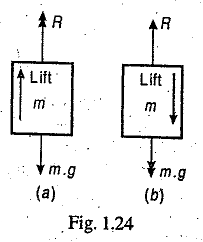# Motion of a Lift or Elevator

Describe Motion of lift or an Elevator. How do we calculate tension in the cable supporting the lift/elevator?

Consider a lift (elevator or cage) carrying some mass and moving with uniform acceleration.

Let
m = Mass carried by the lift in kg,
a = Uniform acceleration of the lift in m/s2, and
R = Reaction of the lift or tension in the cable supporting the lift in newtons.When the lift is moving upwards as shown in Fig. 1.24 (a), then

R – m.g = m.a or R = m.g + m.a = m (g + a)

When the lift is moving downwards as shown in Fig. 1.24 (b), then

m.g – R = m.a or R = m.g – m.a = m (g – a)